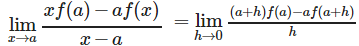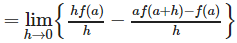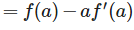Courses

# Test: Continuity And Differentiability (CBSE Level) - 2

## 25 Questions MCQ Test Mathematics (Maths) Class 12 | Test: Continuity And Differentiability (CBSE Level) - 2

Description
This mock test of Test: Continuity And Differentiability (CBSE Level) - 2 for JEE helps you for every JEE entrance exam. This contains 25 Multiple Choice Questions for JEE Test: Continuity And Differentiability (CBSE Level) - 2 (mcq) to study with solutions a complete question bank. The solved questions answers in this Test: Continuity And Differentiability (CBSE Level) - 2 quiz give you a good mix of easy questions and tough questions. JEE students definitely take this Test: Continuity And Differentiability (CBSE Level) - 2 exercise for a better result in the exam. You can find other Test: Continuity And Differentiability (CBSE Level) - 2 extra questions, long questions & short questions for JEE on EduRev as well by searching above.
QUESTION: 1

###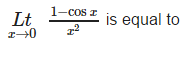Solution:

L'Hopital's rules says that the
lim x→a f(x)/g(x)
⇒ f'(a)/g'(a)
Using this, we get
lim x→0 (1−cosx)/x2
⇒ − sin0/2(0)
Yet as the denominator is 0, this is impossible. So we do a second limit:
lim(x→0) sinx/2x
⇒ cos0/2 = 1/2 = 0.5
So, in total lim x→0 (1−cosx)/x2
⇒ lim x→0 sinx/2x
⇒ cosx/2
⇒ cos0/2= 1/2

QUESTION: 2

### If f (x) is a polynomial of degree m (⩾1) , then which of the following is not true ?

Solution:

As all the three remaining statements are true for the given function.

QUESTION: 3

### Let f and g be differentiable functions such that fog = I, the identity function. If g’ (a) = 2 and g (a) = b, then f ‘ (b) =

Solution:

f(g(x)) = x
f'(g(x)) g'(x) = 1
put x = a
f'(b) g'(a) = 1
2 f'(b) = 1
f'(b) = 1/2

QUESTION: 4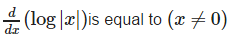Solution: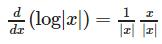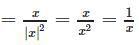QUESTION: 5

If f (x) =x2g(x) and g (x) is twice differentiable then f’’’ (x) is equal to

Solution: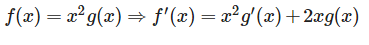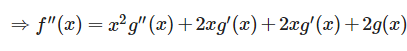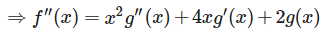QUESTION: 6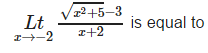Solution: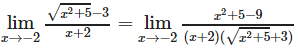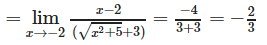QUESTION: 7

If f(x) = | x | ∀ x ∈ R, then

Solution: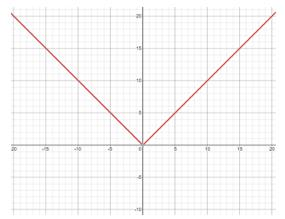The graph of f(x) = |x|
As observed from the graph, f(x) = |x| is continuous at x = 0.
As this curve is pointed at x = 0, f(x) is not derivable at x = 0.

QUESTION: 8

Differential coefficient of a function f (g (x)) w.r.t. the function g (x) is

Solution: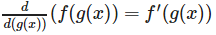QUESTION: 9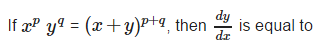Solution:

Given xpyq = (x+y)p+qTaking log on both sides we get:
plogx+qlogy = (p+q) log (x+y).  Differentiating both sides w.r.t. x we get ,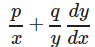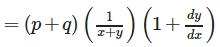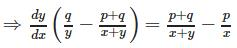QUESTION: 10

If y = aemx + be−mx, then y2 is equal to

Solution:

y = aemx + be-mx ⇒ y1 = amemx + (-m)be-mx ⇒y2

= am2emx + (m2)be-mx ⇒y2 = m2 (aemx + be-mx) ⇒ y2 = m2y

QUESTION: 11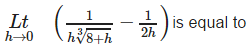Solution: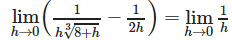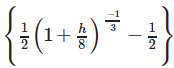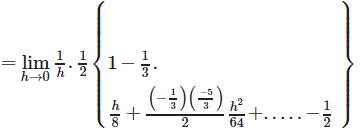QUESTION: 12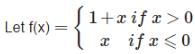then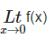is equal to

Solution:
QUESTION: 13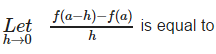Solution: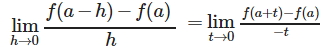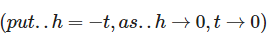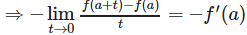QUESTION: 14

If f(x) be any function which assumes only positive values and f’ (x) exists then f’ (x) is equal to

Solution: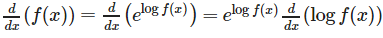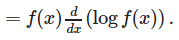QUESTION: 15

If y = a sin mx + b cos m x, then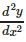is equal to

Solution:

y  = a sin mx+b cos mx ⇒ y1 = am cos mx − bm sin mx
⇒ y2 = −am2sin mx−bm2cosmx ⇒ y2 = −m2(a sin mx+b cos mx)

QUESTION: 16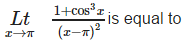Solution: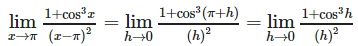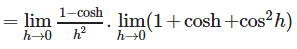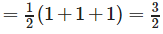QUESTION: 17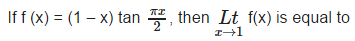Solution: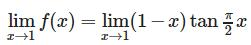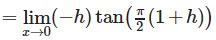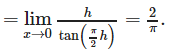QUESTION: 18

If y = tan−1x and z = cot−1x then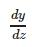is equal to

Solution: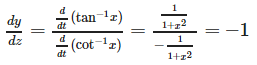QUESTION: 19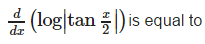Solution: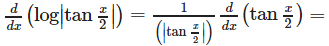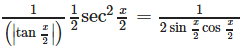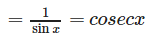QUESTION: 20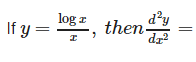Solution: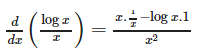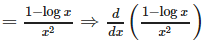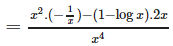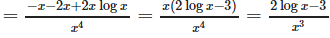QUESTION: 21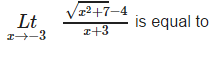Solution: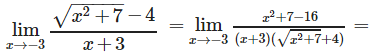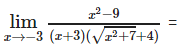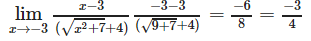QUESTION: 22

If both f and g are defined in a nhd of 0 ; f(0) = 0 = g(0) and f ‘ (0) = 8 = g’ (0), then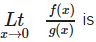equal to

Solution: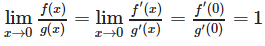(by using L’Hospital Rule)

QUESTION: 23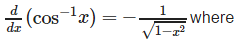Solution: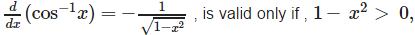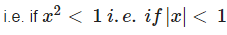QUESTION: 24

The differential coefficient of log (| log x |) w.r.t. log x is

Solution: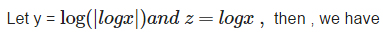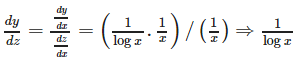QUESTION: 25

If f is derivable at x = a , then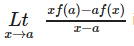is equal to

Solution: